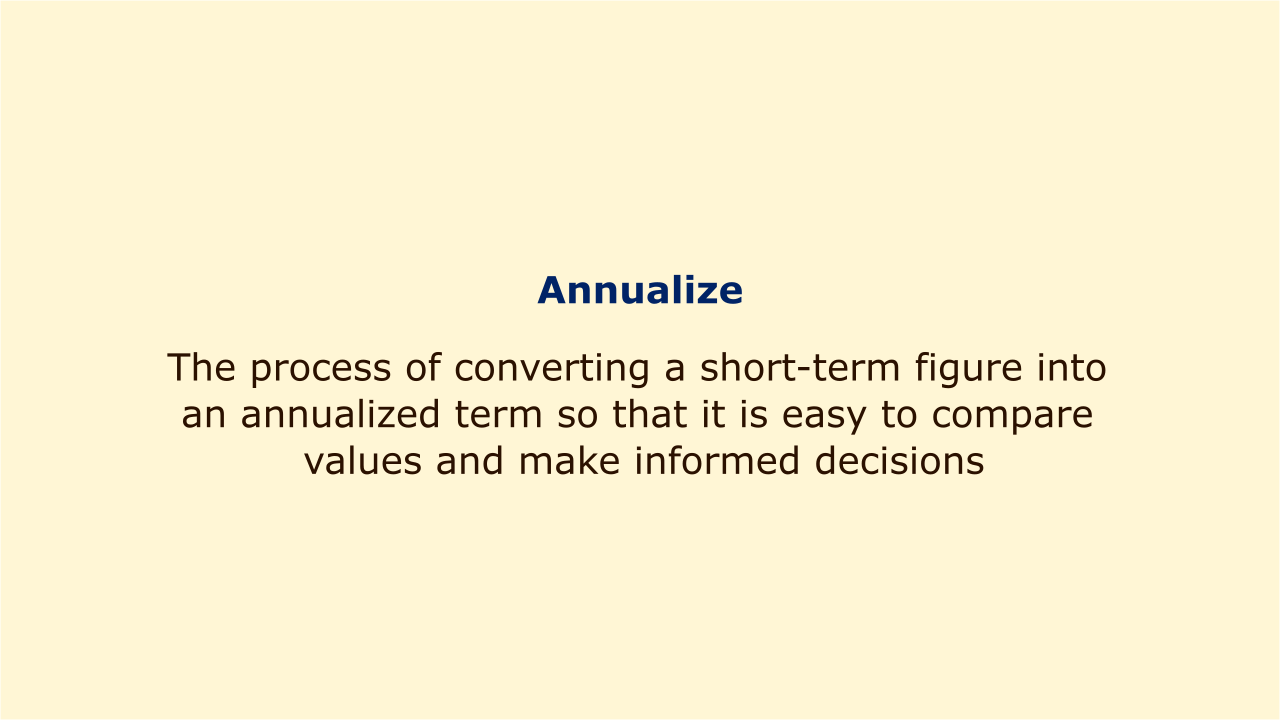# AnnualizeImage: Moneybestpal.com

### To annualize is the process of converting a short-term figure into an annualized term so that it is easy to compare values and make informed decisions. Investors, borrowers, and companies who want to assess the efficiency or cost of various assets, securities, or loans can benefit from annualizing.

Investors can compare the returns of various assets with various time periods by annualizing. When comparing, for instance, a three-month Treasury bill with a 0.5% yield with a one-year bond with a 2% yield, investors can annualize the Treasury bill's return using the following formula:

Annualized return = (1 + periodic return) ^ (number of periods in a year) - 1

The annualized return on the Treasury bill in this situation is (1 + 0.005) 4 - 1 = 0.0201 or 2.01%. This indicates that if the investor reinvested the Treasury bill's principal and interest at the same rate every three months, they would have earned 2.01% in a full year. Investor may see that the Treasury bill delivers a slightly greater return by contrasting this with the bond's yield of 2%.

By annualizing, borrowers can better comprehend the full cost of borrowing money, including any fees and costs not already accounted for in the interest rate. The annual percentage rate (APR), which accounts for both interest and fees, is the cost of borrowing annualized. For example, if a borrower takes out a \$10,000 loan for six months at an interest rate of 12% and pays a \$200 origination fee, the APR is calculated as follows:

APR = [(total interest + fees) / loan amount] / (loan term in years)

In this instance, [(600 + 200) / 10,000] / 0.5 = 0.16, or 16%, is the APR. In other words, over the course of a year, the borrower pays interest and fees totaling 16% of the loan amount.

Businesses can compare the cost of owning or operating various assets over the course of their existence by annualizing. The annualized cost of owning an asset that includes both the initial asset purchase price and ongoing operational expenses is known as the equivalent annual cost (EAC). For example, if a company wants to compare two machines that have different lifespans and operating costs, they can use the EAC formula:

EAC = (initial cost x annuity factor) + annual operating cost

The annuity factor is calculated as:

Annuity factor = [interest rate / (1 - (1 + interest rate) ^ (-number of years))]

Assume that Machine A, which costs \$10,000 and lasts for five years at a cost of \$2,000 per year, and Machine B, which costs \$15,000 and lasts for seven years at a cost of \$1,500 per year each. Assuming an interest rate of 10%, the EACs of both machines are:

EAC of machine A = (10,000 x 0.2638) + 2,000 = \$4,638

EAC of machine B = (15,000 x 0.2122) + 1,500 = \$4,683

This means that Machine A has a lower annualized cost than Machine B over their lifetimes.
Tags